Home | | Physics 12th Std | Ampere’s Circuital Law

# Ampere’s Circuital Law

Ampère’s circuital law is used to calculate magnetic field at a point whenever there is a symmetry in the problem.

AMPERE’S CIRCUITAL LAW

Ampère’s circuital law is used to calculate magnetic field at a point whenever there is a symmetry in the problem. This is similar to Gauss’s law in electrostatics. These are powerful methods whenever there is symmetry in the problem.

## 1. Definition andexplanation of Ampère’s circuital law

Ampère’s law: The line integral of magnetic field over a closed loop is μ0 times net current enclosed by the loop.where Ienclosed is the net current linked by the closed loop C. Note that the line integral does not depend on the shape of the path or the position of the conductor with the magnetic field.

## 2. Magnetic field due tothe current carrying wire of infinite length using Ampère’s lawConsider a straight conductor of infinite length carrying current I and the direction ofmagnetic field lines is shown in Figure 3.42. Since the wire is geometrically cylindrical in shape and symmetrical about its axis, we construct an Ampèrian loop in the form of a circular shape at a distance r from the centre of the conductor as shown in Figure 3.42. From the Ampère’s law, we get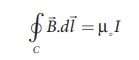whereis the line element along the amperian loop (tangent to the circular loop). Hence, the angle between magnetic field vector and line element is zero. Therefore,where I is the current enclosed by the Ampèrian loop. Due to the symmetry, the magnitude of the magnetic field is uniform over the Ampèrian loop, we can take B out of the integration.For a circular loop, the circumference is 2πr, which implies,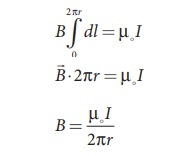In vector form, the magnetic field is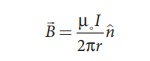where n is the unit vector along the tangent to the Ampèrian loop as shown in the Figure 3.42. This perfectly agrees with the result obtained from Biot-Savart’s law as given in equation (3.39).

EXAMPLE 3.18

Compute the magnitude of the magnetic field of a long, straight wire carrying a current of 1A at distance of 1m from it. Compare it with Earth’s magnetic field.

Solution

Given that 1 = 1 A and radius r = 1 m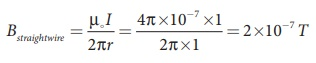But the Earth’s magnetic field is BEarth  ≈ 10−5 T

So, Bstraightwire is one hundred times smaller than BEarth.

## 3. Solenoid

A solenoid is a long coil of wire closely wound in the form of helix as shown in Figure 3.43. When electric current is passed through the solenoid, the magnetic field is produced. The magnetic field of the solenoid is due to the superposition of magnetic fields of each turn of the solenoid. The direction of magnetic field due to solenoid is given by right hand palm-rule (mnemonic).

Inside the solenoid, the magnetic field is nearly uniform and parallel to its axis whereas, outside the solenoid the field is negligibly small. Based on the direction of the current, one end of the solenoid behaves like North Pole and the other end behaves like South Pole.The current carrying solenoid is held in right hand. If the fingers curl in the direction of current, then extended thumb gives the direction of magnetic field of current carrying solenoid. It is shown in Figure 3.44. Hence, the magnetic field of a solenoid looks like the magnetic field of a bar magnet.The solenoid is assumed to be long which means that the length of the solenoid is large when compared to its diameter. The winding need not to be always circular, it can also be in other shapes. We consider here only circularly wound solenoid as shown in Figure 3.45.## 4. Magnetic field due to along current carrying solenoid

Consider a solenoid of length L having N turns. The diametre of the solenoid is assumed to be much smaller when compared to its length and the coil is wound very closely.In order to calculate the magnetic field at any point inside the solenoid, we use Ampere’s circuital law. Consider a rectangular loop abcd as shown in Figure 3.46. Then from Ampère’s circuital law,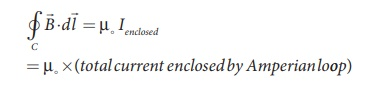The left hand side of the equation is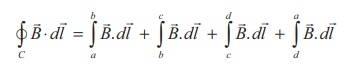Since the elemental lengths along bc and da are perpendicular to the magnetic field which is along the axis of the solenoid, the integralsSince the magnetic field outside the solenoid is zero, the integralFor the path along ab, the integral iswhere the length of the loop ab as shown in the Figure 3.46 is h. But the choice of length of the loop ab is arbitrary. We can take very large loop such that it is equal to the length of the solenoid L. Therefore the integral is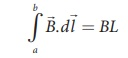Let N I be the current passing through the solenoid of N turns, thenThe number of turns per unit length is given by N/L = n, Then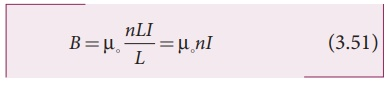Since n is a constant for a given solenoid and μ0 is also constant. For a fixed current I, the magnetic field inside the solenoid is also a constant.

EXAMPLE 3.19

Calculate the magnetic field inside a solenoid, when

(a) the length of the solenoid becomes twice and fixed number of turns

(b) both the length of the solenoid and number of turns are double

(c) the number of turns becomes twice for the fixed length of the solenoid

Compare the results.

Solution

The magnetic field of a solenoid (inside) is(a) length of the solenoid becomes twice and fixed number of turns

L→2L (length becomes twice)

N→N (number of turns are fixed)

The magnetic field is

B2L , N = µ NI/2L = 1/2 BL ,N

(b) both the length of the solenoid and number of turns are double

L→2L (length becomes twice)

N→2N (number of turns becomes twice)

The magnetic field is(c) the number of turns becomes twice but for the fixed length of the solenoid

L→L (length is fixed)

N→2N (number of turns becomes twice)

The magnetic field is

BL ,2 N = µ, 2NI/L = 2BL ,N

From the above results,

BL ,2 N > B2 L ,2 N > B2 L , N

Thus, strength of the magnetic field is increased when we pack more loops into the same length for a given current.

## 5. Toroid

A solenoid is bent in such a way its ends are joined together to form a closed ring shape, is called a toroid which is shown in Figure 3.47. The magnetic field has constant magnitude inside the toroid whereas in the interior region (say, at point P) and exterior region (say, at point Q), the magnetic field is zero.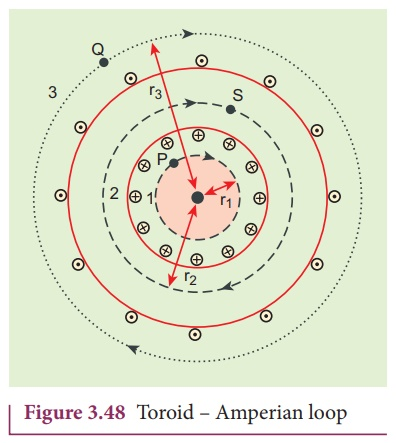### (a) Open space interior to the toroid

Let us calculate the magnetic field BP at point P. We construct an Amperian loop 1 of radius r1 around the point P as shown in Figure 3.48. For simplicity, we take circular loop so that the length of the loop is its circumference.

L1 = 2πr1

Ampère’s circuital law for the loop 1 isSince, the loop1 encloses no current, Ienclosed = 0This is possible only if the magnetic field at point P vanishes i.e.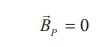### (b) Open space exterior to the toroid

Let us calculate the magnetic field BQ at point Q. We construct an Amperian loop 3 of radius r3 around the point Q as shown in Figure 3.48. The length of the loop isL3 = r3

Ampère’s circuital law for the loop 3 is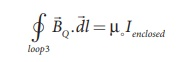Since, in each turn of the toroid loop, current coming out of the plane of paper is cancelled by the current going into the plane of paper. Thus, Ienclosed = 0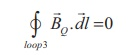This is possible only if the magnetic field at point Q vanishes i.e.### (c) Inside the toroid

Let us calculate the magnetic field BS at point S by constructing an Amperian loop 2 of radius r2 around the point S as shown in Figure 3.48. The length of the loop is

L2 = r2

Ampere’s circuital law for the loop 2 isLet I be the current passing through the toroid and N be the number of turns of the toroid, thenThe number of turns per unit length is n = N/2πr2 , then the magnetic field at point S isStudy Material, Lecturing Notes, Assignment, Reference, Wiki description explanation, brief detail
12th Physics : Magnetism and Magnetic Effects of Electric Current : Ampere’s Circuital Law |